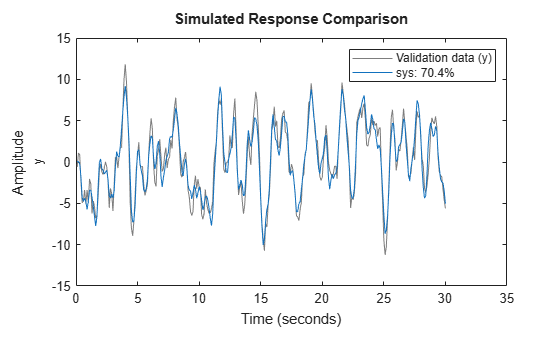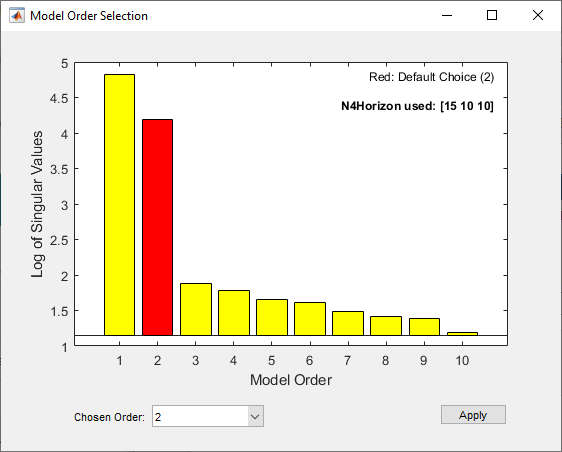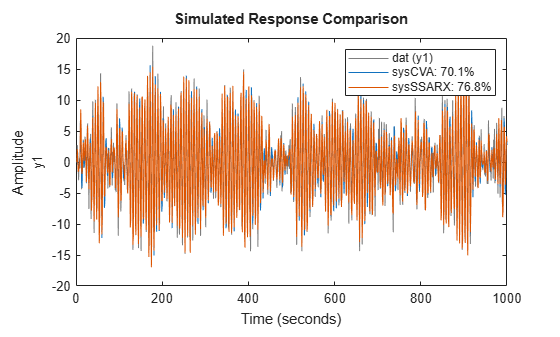# n4sid

Estimate state-space model using subspace method with time-domain or frequency-domain data

## Syntax

``sys = n4sid(data,nx)``
``sys = n4sid(data,nx,Name,Value)``
``sys = n4sid(___,opt)``
``[sys,x0] = n4sid(___)``

## Description

example

````sys = n4sid(data,nx)` estimates a discrete-time state-space model `sys` of order `nx` using `data`, which can be time-domain or frequency-domain data. `sys` is a model of the following form:$\begin{array}{l}\stackrel{˙}{x}\left(t\right)=Ax\left(t\right)+Bu\left(t\right)+Ke\left(t\right)\\ y\left(t\right)=Cx\left(t\right)+Du\left(t\right)+e\left(t\right)\end{array}$A, B, C, D, and K are state-space matrices. u(t) is the input, y(t) is the output, e(t) is the disturbance, and x(t) is the vector of `nx` states.All entries of A, B, C, and K are free estimable parameters by default. For dynamic systems, D is fixed to zero by default, meaning that the system has no feedthrough. For static systems (`nx = 0`), D is an estimable parameter by default.```

example

````sys = n4sid(data,nx,Name,Value)` incorporates additional options specified by one or more name-value pair arguments. For example, to estimate a continuous-time model, specify the sample time `'Ts'` as `0`. Use the `'Form'`, `'Feedthrough'`, and `'DisturbanceModel'` name-value pair arguments to modify the default behavior of the A, B, C, D, and K matrices.```

example

````sys = n4sid(___,opt)` specifies the estimation options `opt`. These options can include the initial states, estimation objective, and subspace algorithm related choices to be used for estimation. Specify `opt` after any of the previous input-argument combinations.```
````[sys,x0] = n4sid(___)` returns the value of initial states computed during estimation. You can use this syntax with any of the previous input-argument combinations.```

## Examples

collapse all

Estimate a state-space model and compare its response with the measured output.

Load the input-output data `z1`, which is stored in an `iddata` object.

`load iddata1 z1`

Estimate a fourth-order state-space model.

```nx = 4; sys = n4sid(z1,nx)```
```sys = Discrete-time identified state-space model: x(t+Ts) = A x(t) + B u(t) + K e(t) y(t) = C x(t) + D u(t) + e(t) A = x1 x2 x3 x4 x1 0.8392 -0.3129 0.02105 0.03743 x2 0.4768 0.6671 0.1428 0.003757 x3 -0.01951 0.08374 -0.09761 1.046 x4 -0.003885 -0.02914 -0.8796 -0.03171 B = u1 x1 0.02635 x2 -0.03301 x3 7.256e-05 x4 0.0005861 C = x1 x2 x3 x4 y1 69.08 26.64 -2.237 -0.5601 D = u1 y1 0 K = y1 x1 0.003282 x2 0.009339 x3 -0.003232 x4 0.003809 Sample time: 0.1 seconds Parameterization: FREE form (all coefficients in A, B, C free). Feedthrough: none Disturbance component: estimate Number of free coefficients: 28 Use "idssdata", "getpvec", "getcov" for parameters and their uncertainties. Status: Estimated using N4SID on time domain data "z1". Fit to estimation data: 76.33% (prediction focus) FPE: 1.21, MSE: 1.087 ```

Compare the simulated model response with the measured output.

`compare(z1,sys)`The plot shows that the fit percentage between the simulated model and the estimation data is greater than 70%.

You can view more information about the estimation by exploring the `idss` property `sys.Report`.

`sys.Report`
```ans = Status: 'Estimated using N4SID with prediction focus' Method: 'N4SID' InitialState: 'estimate' N4Weight: 'CVA' N4Horizon: [6 10 10] Fit: [1x1 struct] Parameters: [1x1 struct] OptionsUsed: [1x1 idoptions.n4sid] RandState: [1x1 struct] DataUsed: [1x1 struct] ```

`sys.Report.Parameters.X0`
```ans = 4×1 -0.0085 0.0052 -0.0193 0.0282 ```

Load the input-output data `z1`, which is stored in an `iddata` object.

`load iddata1 z1`

Determine the optimal model order by specifying argument `nx` as a range from 1 to 10.

```nx = 1:10; sys = n4sid(z1,nx);```

An automatically generated plot shows the Hankel singular values for models of the orders specified by `nx`.States with relatively small Hankel singular values can be safely discarded. The suggested default order choice is `2`.

Select the model order in the Chosen Order list and click .

`load iddata2 z2`

Specify estimation options. Set the weighting scheme `'N4Weight'` to `'SSARX'` and estimation-status display option `'Display'` to `'on'`.

`opt = n4sidOptions('N4Weight','SSARX','Display','on')`
```Option set for the n4sid command: InitialState: 'estimate' N4Weight: 'SSARX' N4Horizon: 'auto' Display: 'on' InputOffset: [] OutputOffset: [] EstimateCovariance: 1 OutputWeight: [] Focus: 'prediction' WeightingFilter: [] EnforceStability: 0 Advanced: [1x1 struct] ```

Estimate a third-order state-space model using the updated option set.

```nx = 3; sys = n4sid(z2,nx,opt);```

Modify the canonical form of the A, B, and C matrices, include a feedthrough term in the D matrix, and eliminate disturbance-model estimation in the K matrix.

Load input-output data and estimate a fourth-order system using the `n4sid` default options.

```load iddata1 z1 sys1 = n4sid(z1,4);```

Specify the modal form and compare the `A` matrix with the default `A` matrix.

```sys2 = n4sid(z1,4,'Form','modal'); A1 = sys1.A```
```A1 = 4×4 0.8392 -0.3129 0.0211 0.0374 0.4768 0.6671 0.1428 0.0038 -0.0195 0.0837 -0.0976 1.0462 -0.0039 -0.0291 -0.8796 -0.0317 ```
`A2 = sys2.A`
```A2 = 4×4 0.7554 0.3779 0 0 -0.3779 0.7554 0 0 0 0 -0.0669 0.9542 0 0 -0.9542 -0.0669 ```

Include a feedthrough term and compare the `D` matrices.

```sys3 = n4sid(z1,4,'Feedthrough',1); D1 = sys1.D```
```D1 = 0 ```
`D3 = sys3.D`
```D3 = 0.0487 ```

Eliminate disturbance modeling and compare the `K` matrices.

```sys4 = n4sid(z1,4,'DisturbanceModel','none'); K1 = sys1.K```
```K1 = 4×1 0.0033 0.0093 -0.0032 0.0038 ```
`K4 = sys4.K`
```K4 = 4×1 0 0 0 0 ```

Estimate a continuous-time canonical-form model.

`load iddata1 z1`

Estimate the model. Set `Ts` to `0` to specify a continuous model.

```nx = 2; sys = n4sid(z1,nx,'Ts',0,'Form','canonical');```

`sys` is a second-order continuous-time state-space model in the canonical form.

Estimate a state-space model from closed-loop data using the subspace algorithm `SSARX`. This algorithm is better at capturing feedback effects than other weighting algorithms.

Generate closed-loop estimation data for a second-order system corrupted by white noise.

```N = 1000; K = 0.5; rng('default'); w = randn(N,1); z = zeros(N,1); u = zeros(N,1); y = zeros(N,1); e = randn(N,1); v = filter([1 0.5],[1 1.5 0.7],e); for k = 3:N u(k-1) = -K*y(k-2) + w(k); u(k-1) = -K*y(k-1) + w(k); z(k) = 1.5*z(k-1) - 0.7*z(k-2) + u(k-1) + 0.5*u(k-2); y(k) = z(k) + 0.8*v(k); end dat = iddata(y, u, 1);```

Specify the weighting scheme `'N4weight'` used by the N4SID algorithm. Create two option sets. For one option set, set `'N4weight'` to `'CVA'`. For the other option set, set the `'N4weight'` to `'SSARX'`.

```optCVA = n4sidOptions('N4weight','CVA'); optSSARX = n4sidOptions('N4weight','SSARX');```

Estimate state-space models using the option sets.

```sysCVA = n4sid(dat,2,optCVA); sysSSARX = n4sid(dat,2,optSSARX);```

Compare the fit of the two models with the estimation data.

`compare(dat,sysCVA,sysSSARX);`As the plot shows, the model estimated using the SSARX algorithm produces a better fit than the model estimated using the CVA algorithm.

## Input Arguments

collapse all

Estimation data, specified as an `iddata` object, an `frd` object, or an `idfrd` object.

For time-domain estimation, `data` must be an `iddata` object containing the input and output signal values.

For frequency-domain estimation, `data` can be one of the following:

• Recorded frequency response data (`frd` (Control System Toolbox) or `idfrd`)

• `iddata` object with properties specified as follows.

• `InputData` — Fourier transform of the input signal

• `OutputData` — Fourier transform of the output signal

• `Domain``'Frequency'`

Estimation data must be uniformly sampled. By default, the software sets the sample time of the model to the sample time of the estimation data.

For multiexperiment data, the sample times and intersample behavior of all the experiments must match.

The domain of your data determines the type of model you can estimate.

• Time-domain or discrete-time frequency-domain data — Continuous-time and discrete-time models

• Continuous-time frequency-domain data — Continuous-time models only

Order of the estimated model, specified as a nonnegative integer or as a vector containing a range of positive integers.

• If you already know what order you want your estimated model to have, specify `nx` as a scalar.

• If you want to compare a range of potential orders to choose the most effective order for your estimated model, specify that range for `nx`. `n4sid` creates a Hankel singular-value plot that shows the relative energy contributions of each state in the system. States with relatively small Hankel singular values contribute little to the accuracy of the model and can be discarded with little impact. The index of the highest state you retain is the model order. The plot window includes a suggestion for the order to use. You can accept this suggestion or enter a different order. For an example, see Determine Optimal Estimated Model Order.

If you do not specify `nx`, or if you specify `nx` as `best`, the software automatically chooses `nx` from the range `1:10`.

• If you are identifying a static system, set `nx` to `0`.

Estimation options, specified as an `n4sidOptions` option set. Options specified by `opt` include:

• Estimation objective

• Handling of initial conditions

• Subspace algorithm-related choices

For examples showing how to specify options, see Specify Estimation Options and Continuous-Time Canonical-Form Model.

### Name-Value Arguments

Specify optional pairs of arguments as `Name1=Value1,...,NameN=ValueN`, where `Name` is the argument name and `Value` is the corresponding value. Name-value arguments must appear after other arguments, but the order of the pairs does not matter.

Before R2021a, use commas to separate each name and value, and enclose `Name` in quotes.

Example: `sys = n4sid(data,nx,'Form','modal')`

Sample time of the estimated model, specified as the comma-separated pair consisting of `'Ts'` and either `0` or a positive scalar.

• For continuous-time models, specify `'Ts'` as `0`. In the frequency domain, using continuous-time frequency-domain data results in a continuous-time model.

• For discrete-time models, the software sets `'Ts'` to the sample time of the data in the units stored in the `TimeUnit` property.

Input delay for each input channel, specified as the comma-separated pair consisting of `'InputDelay'` and a numeric vector.

• For continuous-time models, specify `'InputDelay'` in the time units stored in the `TimeUnit` property.

• For discrete-time models, specify `'InputDelay'` in integer multiples of the sample time `Ts`. For example, setting `'InputDelay'` to `3` specifies a delay of three sampling periods.

For a system with Nu inputs, set `InputDelay` to an Nu-by-1 vector. Each entry of this vector is a numerical value that represents the input delay for the corresponding input channel.

To apply the same delay to all channels, specify `'InputDelay'` as a scalar.

Type of canonical form of `sys`, specified as the comma-separated pair consisting of `'Form'` and one of the following values:

• `'free'` — Treat all entries of the matrices A, B, C, D, and K as free.

• `'modal'` — Obtain `sys` in modal form.

• `'companion'` — Obtain `sys` in companion form.

• `'canonical'` — Obtain `sys` in the observability canonical form.

For definitions of the canonical forms, see Canonical State-Space Realizations.

For an example, see Modify Form, Feedthrough, and Disturbance-Model Matrices.

Direct feedthrough from input to output, specified as the comma-separated pair consisting of `'Feedthrough'` and a logical vector of length Nu, where Nu is the number of inputs. If you specify `'Feedthrough'` as a logical scalar, that value is applied to all the inputs. For static systems, the software always assumes `'Feedthrough'` is `1`.

For an example, see Modify Form, Feedthrough, and Disturbance-Model Matrices.

Option to estimate time-domain noise component parameters in the K matrix, specified as the comma-separated pair consisting of `'DisturbanceModel'` and one of the following values:

• `'estimate'` — Estimate the noise component. The K matrix is treated as a free parameter. For time-domain data, `'estimate'` is the default.

• `'none'` — Do not estimate the noise component. The elements of the K matrix are fixed at zero. For frequency-domain data, `'none'` is the default and the only acceptable value.

For an example, see Modify Form, Feedthrough, and Disturbance-Model Matrices.

## Output Arguments

collapse all

Identified state-space model, returned as an `idss` model. This model is created using the specified model orders, delays, and estimation options.

Information about the estimation results and options used is stored in the `Report` property of the model. `Report` has the following fields.

Report FieldDescription
`Status`

Summary of the model status, which indicates whether the model was created by construction or obtained by estimation.

`Method`

Estimation command used.

`InitialState`

How initial states were handled during estimation, returned as one of the following values:

• `'zero'` — The initial state is set to zero.

• `'estimate'` — The initial state is treated as an independent estimation parameter.

This field is especially useful when the `'InitialState'` option in the estimation option set is `'auto'`.

`N4Weight`

Weighting scheme used for singular-value decomposition by the N4SID algorithm, returned as one of the following values:

• `'MOESP'` — Uses the MOESP algorithm.

• `'CVA'` — Uses the Canonical Variate Algorithm.

• `'SSARX'` — A subspace identification method that uses an ARX estimation-based algorithm to compute the weighting.

This option is especially useful when the `N4Weight` option in the estimation option set is `'auto'`.

`N4Horizon`

Forward and backward prediction horizons used by the N4SID algorithm, returned as a row vector with three elements ` [r sy su]`, where `r` is the maximum forward prediction horizon, `sy` is the number of past outputs, and `su` is the number of past inputs that are used for the predictions.

`Fit`

Quantitative assessment of the estimation, returned as a structure. See Loss Function and Model Quality Metrics for more information on these quality metrics. The structure has the following fields:

FieldDescription
`FitPercent`

Normalized root mean squared error (NRMSE) measure of how well the response of the model fits the estimation data, expressed as the percentage fitpercent = 100(1-NRMSE).

`LossFcn`

Value of the loss function when the estimation completes.

`MSE`

Mean squared error (MSE) measure of how well the response of the model fits the estimation data.

`FPE`

Final prediction error for the model.

`AIC`

Raw Akaike Information Criteria (AIC) measure of model quality.

`AICc`

Small-sample-size corrected AIC.

`nAIC`

Normalized AIC.

`BIC`

Bayesian Information Criteria (BIC).

`Parameters`

Estimated values of model parameters.

`OptionsUsed`

Option set used for estimation. If you did not configure any custom options, `OptionsUsed` is the set of default options. See `n4sidOptions` for more information.

`RandState`

State of the random number stream at the start of estimation. Empty, `[]`, if randomization was not used during estimation. For more information, see `rng`.

`DataUsed`

Attributes of the data used for estimation, returned as a structure with the following fields.

FieldDescription
`Name`

Name of the data set.

`Type`

Data type.

`Length`

Number of data samples.

`Ts`

Sample time.

`InterSample`

Input intersample behavior, returned as one of the following values:

• `'zoh'` — Zero-order hold maintains a piecewise-constant input signal between samples.

• `'foh'` — First-order hold maintains a piecewise-linear input signal between samples.

• `'bl'` — Band-limited behavior specifies that the continuous-time input signal has zero power above the Nyquist frequency.

`InputOffset`

Offset removed from time-domain input data during estimation. For nonlinear models, it is `[]`.

`OutputOffset`

Offset removed from time-domain output data during estimation. For nonlinear models, it is `[]`.

For more information on using `Report`, see Estimation Report.

Initial states computed during the estimation, returned as an array containing a column vector corresponding to each experiment.

This array is also stored in the `Parameters` field of the model `Report` property.

 Ljung, L. System Identification: Theory for the User, Appendix 4A, Second Edition, pp. 132–134. Upper Saddle River, NJ: Prentice Hall PTR, 1999.

 van Overschee, P., and B. De Moor. Subspace Identification of Linear Systems: Theory, Implementation, Applications. Springer Publishing: 1996.

 Verhaegen, M. "Identification of the deterministic part of MIMO state space models." Automatica, 1994, Vol. 30, pp. 61–74.

 Larimore, W.E. "Canonical variate analysis in identification, filtering and adaptive control." Proceedings of the 29th IEEE Conference on Decision and Control, 1990, pp. 596–604.

 McKelvey, T., H. Akcay, and L. Ljung. "Subspace-based multivariable system identification from frequency response data." IEEE Transactions on Automatic Control, 1996, Vol. 41, pp. 960–979.